# help with C++ code The aim of this is to practice writing template classes. You must...

help with C++ code

The aim of this is to practice writing template classes. You must use SafeArray template class and implement a SafeMatrix template class that will allow you to work with two dimensional arrays of any type. The boundaries are checked. You must be able to create instances of SafeMatrix template class like,

SafeMatrix<int> m(2,3);

The above statement will create a 2 by 3 dimensional array of integers. You must be able to access and set values using bracket operators. For example m = 5; will set element at row 0 column 1 to an integer value 5. You must be able to return dimensions of the array as follows: m.length() will return the number of rows in your 2D array. m.length() will return number of columns in row number 0. Provide all necessary methods to your template class. Write a Driver program that tests your template class with at least two different types.

CODE TO COPY:

#include<iostream>

#define SIZE 500

using namespace std;

//safearray class

template<typename t>

class SafeArray

{

private:

t array[SIZE];

int cols;

public:

SafeArray(int cols)

{//parameterized constructors

this->cols=cols;

//if size is smaller, throw an error

if(SIZE<cols)

{

cout<<"Array Size Overfow!!"<<endl;

return;

}

//initialize the values to 0

for(int i=0; i<cols; i++)

array[i]=0;

}

t& operator[](int y)

{

return array[y];

}

//return cols in the safearray

int length()

{

return cols;

}

friend ostream& operator<<(ostream &out, t& valRef)

{

t val=valRef;

out<<val;

return out;

}

};

//safematrix class

template <typename t>

class SafeMatrix{

private:

SafeArray<t> *matrix[SIZE];

int rows;

int cols;

public:

//parameterized constructor

SafeMatrix(int rows, int cols)

{

this->rows=rows;//set rows

this->cols=cols; //set columns

if(rows>SIZE)//if size is smaller than rows

{

cout<<"Array Index Overflow!!"<<endl;//throw an error

return;//return

}

//initialize the safearrays

for(int i=0; i<rows; i++)

matrix[i]=new SafeArray<t>(cols);

}

//return length of the matrix

int length()

{

return rows;

}

SafeArray<t>& operator[](int x)

{

return *matrix[x];//return pointer to SafeArray

}

};

int main()

{

SafeMatrix<int> sm(3,3);//create a safe matrix of size 3 by 3

sm=5;//set vale at index 0,1 as 5

cout<<"sm.length() -> "<<sm.length()<<endl;//output length of matrix

cout<<"sm.length() --> "<<sm.length()<<endl; //output length of first row of matrix

cout<<"Value of sm -> "<<sm<<endl;//output value at 0,1

cin.get();//hold the colsole screen

return 0;

}

PROGRAM SCREENSHOTS: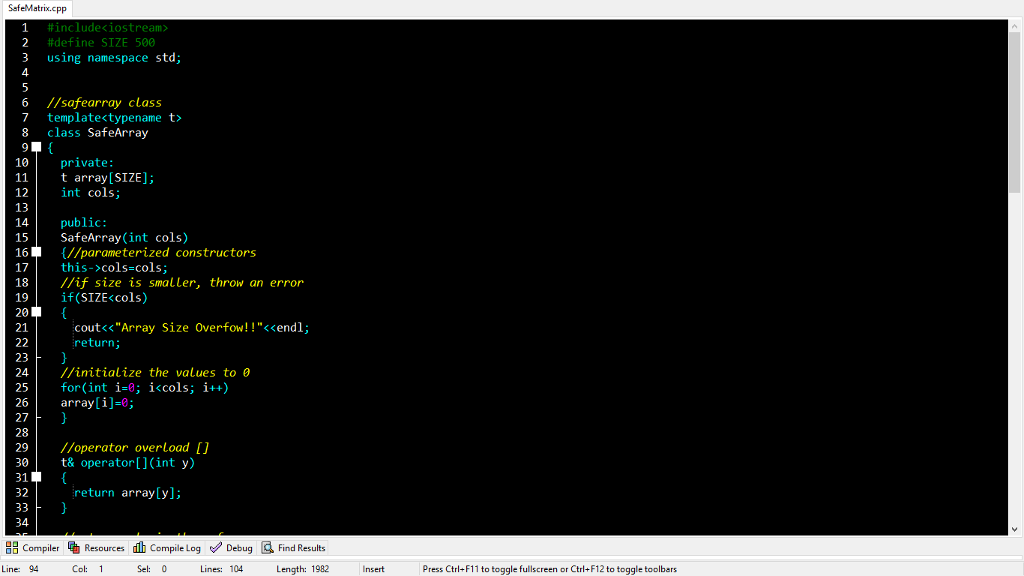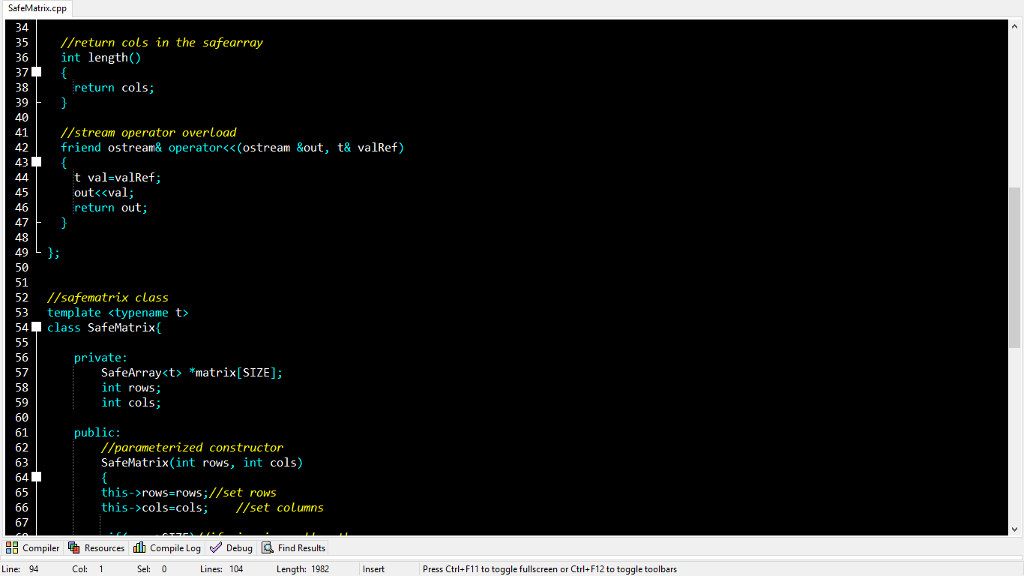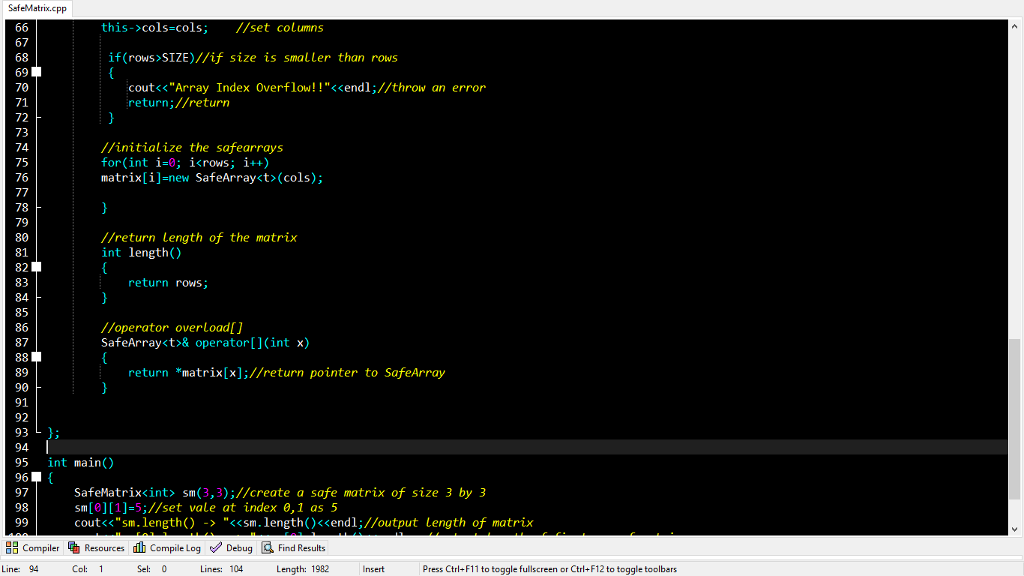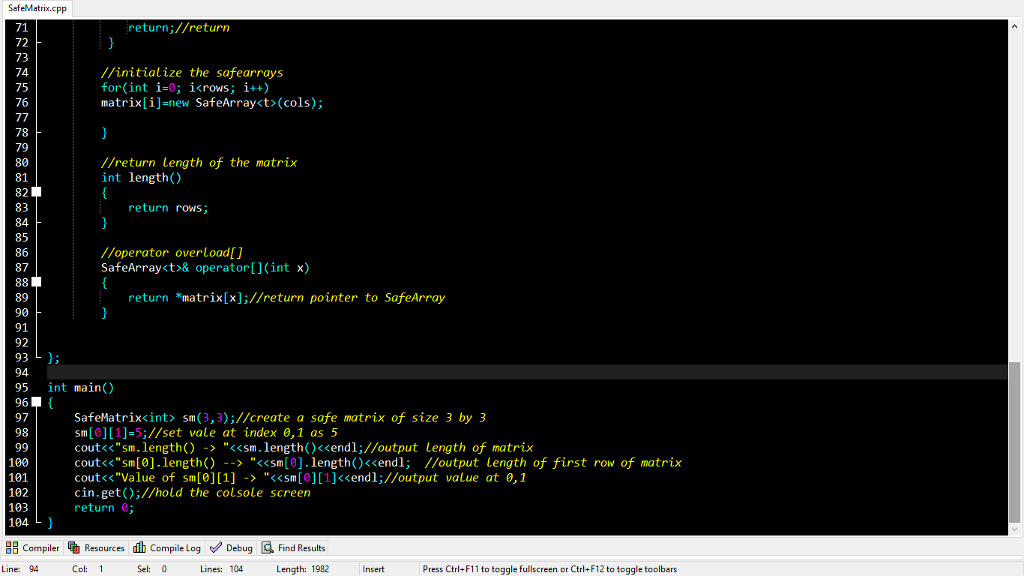OUTPUT: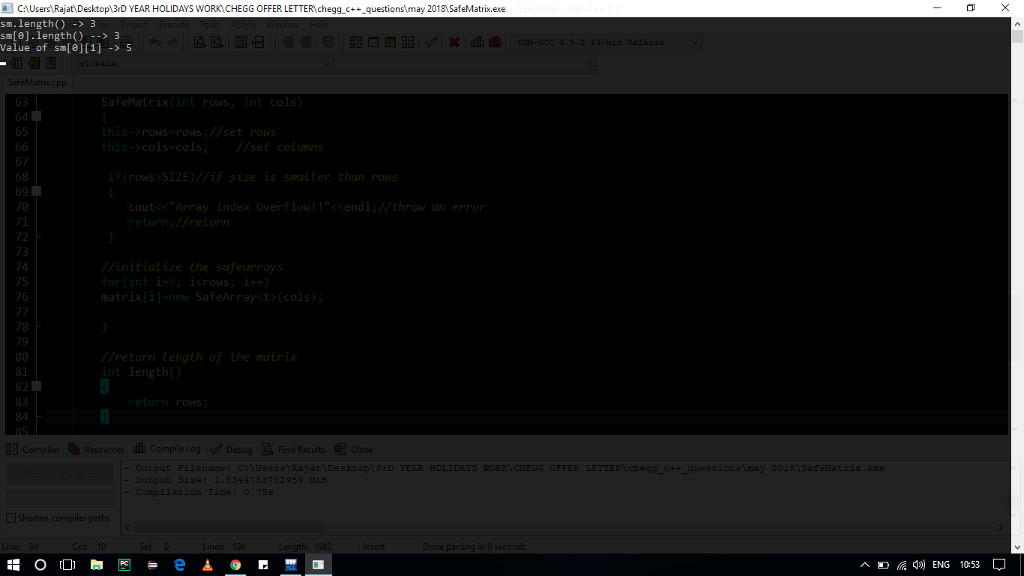In case of any doubt, please let me know in the comments section. I'll get it resolved :) :) Don't forget to give a THUMBS UP!! :)

#### Earn Coin

Coins can be redeemed for fabulous gifts.

Similar Homework Help Questions
• ### Java Here is the template public class ShiftNumbers { public static void main(String[] args) { // TODO:...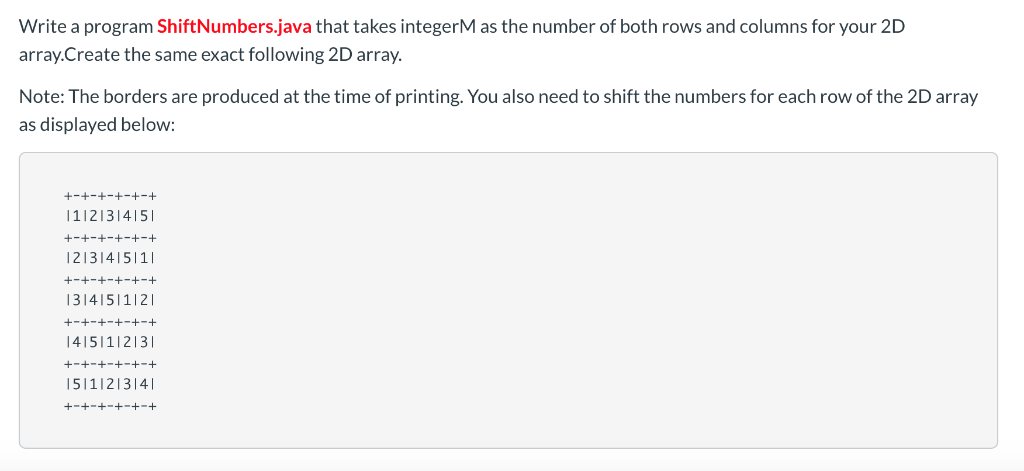Java Here is the template public class ShiftNumbers { public static void main(String[] args) { // TODO: Declare matrix shell size // TODO: Create first row // TODO: Generate remaining rows // TODO: Display matrix } /** * firstRow * * This will generate the first row of the matrix, given the size n. The * elements of this row will be the values from 1 to n * * @param size int Desired size of the array * @return...

• ### Need help with 2D Array Script

public static double[][] trainNetwork(double[][] trainingSet,double[][] kernelSet, double variance, int nClassifications, double learningRate, int nIterations, Random rng){//Create a new 2D array of weights//number of rows: the number of classifications//number of cols: one larger than the number of rows in the KernelSet//Initialize your new weights array with random doubles in the range//-1.0 inclusive to +1.0 exclusive//Create a new 2D array of inputs for the perceptrons//number of rows: the length of the training set//number of cols: same as the number of cols in...

• ### in java / You will: // 1- create a square 2 dimensional array of random size...

in java / You will: // 1- create a square 2 dimensional array of random size (less than 11) // 2- fill the array with random integers from 1 to the size limit // 3- print the array // 4- prompt to see if the user wants to do another //+++++++++++++++++++++++++++++++++++++++++++++++++++++++++++++++++ // 1- The square can use the same random value for x and y. Don't allow 0 size arrays. // 2- Maybe a nested set of for loops? to...

• ### Chapter 8 Exercise 36, Introduction to Java Programming, Tenth Edition Y. Daniel LiangY. 8.36 (Latin square)...

Chapter 8 Exercise 36, Introduction to Java Programming, Tenth Edition Y. Daniel LiangY. 8.36 (Latin square) A Latin square is an n-by-n array filled with n different Latin letters, each occurring exactly once in each row and once in each column. Write a program that prompts the user to enter the number n and the array of characters, as shown in the sample output, and checks if the input array is a Latin square. The characters are the first n...

• ### You are to write a banking application in c# that keeps track of bank accounts. It will consist of three classes, Account, Bank and BankTest. The Account class is used to represent a savings account i...

You are to write a banking application in c# that keeps track of bank accounts. It will consist of three classes, Account, Bank and BankTest. The Account class is used to represent a savings account in a bank. The class must have the following instance variables: a String called accountID                       a String called accountName a two-dimensional integer array called deposits (each row represents deposits made in a week). At this point it should not be given any initial value. Each...

• ### Program in C++! Thank you in advance! Write a menu based program implementing the following functions: (1) Write a funct...

Program in C++! Thank you in advance! Write a menu based program implementing the following functions: (1) Write a function that prompts the user for the name of a file to output as a text file that will hold a two dimensional array of the long double data type. Have the user specify the number of rows and the number of columns for the two dimensional array. Have the user enter the values for each row and column element in...

• ### /** * Class that defines a method which performs matrix addition. This gives students their first...

/** * Class that defines a method which performs matrix addition. This gives students their first practice at creating and using * the code needed for higher dimensional arrays. * * @author Matthew Hertz */ public class MatrixAddition { /** * Given two equally-sized matrices, return a newly allocated matrix containing the result of adding the two.<br/> * Precondition: a &amp; b must have the same number of rows and each row will have the same number of columns. You...

• ### COMP1410 - Lab Exercises #3 (Due at the end of the lab period or beginning of...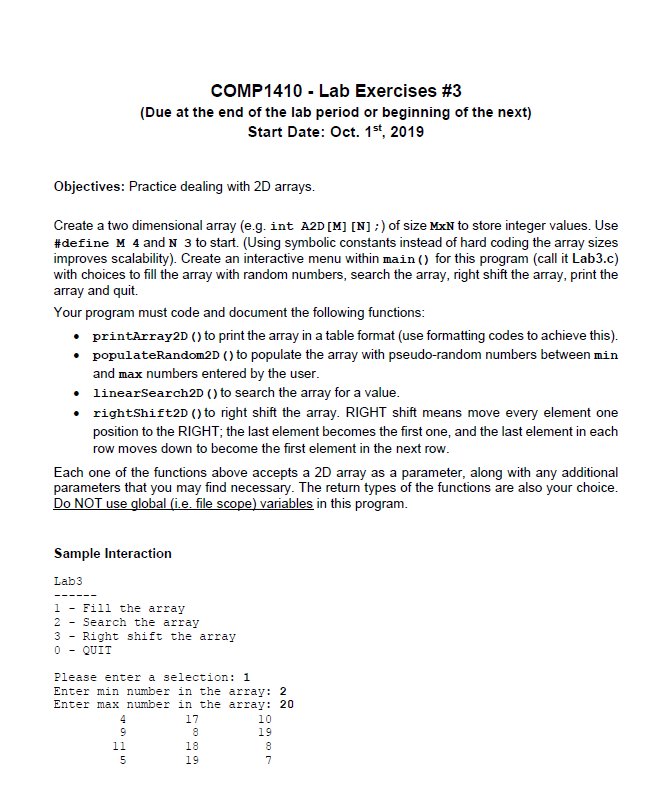COMP1410 - Lab Exercises #3 (Due at the end of the lab period or beginning of the next) Start Date: Oct. 1st, 2019 Objectives: Practice dealing with 2D arrays. Create a two dimensional array (e.g. int A2D[M] [N] ;) of size MXN to store integer values. Use #define M 4 and N 3 to start. (Using symbolic constants instead of hard coding the array sizes improves scalability). Create an interactive menu within main() for this program (call it Lab3.c) with...

• ### Please write below code in C++ using Visual Studio. Write program that uses a class template...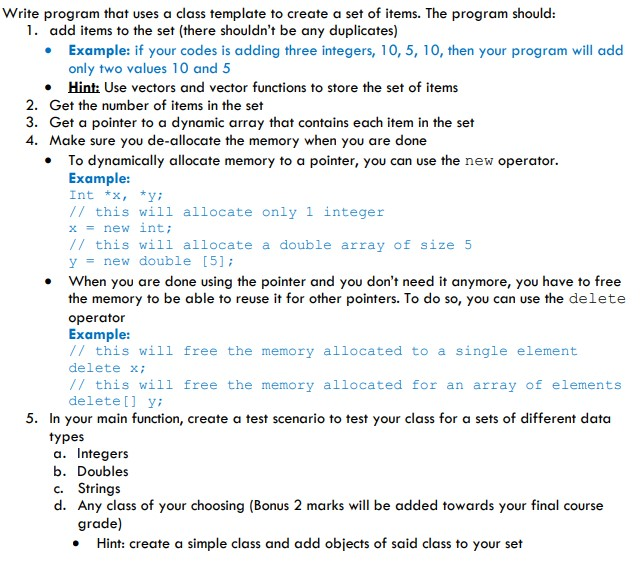Please write below code in C++ using Visual Studio. Write program that uses a class template to create a set of items. The program should: 1. add items to the set (there shouldn't be any duplicates) • Example: if your codes is adding three integers, 10, 5, 10, then your program will add only two values 10 and 5 • Hint: Use vectors and vector functions to store the set of items 2. Get the number of items in the...

• ### Using java : In this exercise, you need to implement a class that encapsulate a Grid. A grid is a useful concept in crea...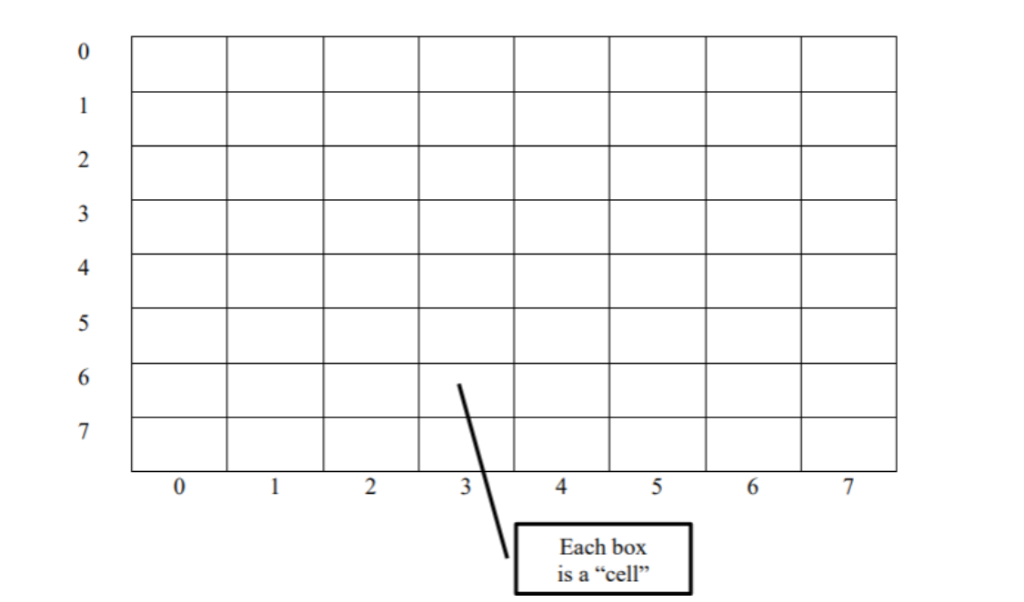Using java : In this exercise, you need to implement a class that encapsulate a Grid. A grid is a useful concept in creating board-game applications. Later we will use this class to create a board game. A grid is a two-dimensional matrix (see example below) with the same number of rows and columns. You can create a grid of size 8, for example, it’s an 8x8 grid. There are 64 cells in this grid. A cell in the grid...

Free Homework App